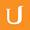## Get to know PDF st095 L6

• 0:00 - 0:05
We can do the same with normal distributions. Which are modeled by a special
• 0:05 - 0:10
probability density function. We're not going to go over the equation for this
• 0:10 - 0:15
probability function in this course, but if you want, you can easily look it up
• 0:15 - 0:20
and see what it is. And that might be pretty cool for some of you that wants a
• 0:20 - 0:24
• 0:24 - 0:28
curve, we can model it with an equation. And then, using this equation, we can
• 0:28 - 0:32
use calculus to find the area under the curve. But we don't need to use
• 0:32 - 0:37
calculus, because someone else already did, and then they created a special
• 0:37 - 0:41
table so that we can always figure out the area under the curve between any two
• 0:41 - 0:47
values. We're going to use this table later first let's make sure we're all up
• 0:47 - 0:52
to speed on the normal probablity density function and the area underneath.
• 0:52 - 0:58
First the tails never actually touch the X axis they get closer and closer to
• 0:58 - 1:03
the X axis so the X axis a horizontal axis. [unknown] the reason the tails of
• 1:03 - 1:09
this theoretical model don't touch the x axis is basically because we can never
• 1:09 - 1:15
be 100% sure of anything, in other words we could have a value way out here
• 1:15 - 1:21
really far from the mean like five standard deviations away But the probability
• 1:21 - 1:27
of getting this value or lower is very small. And it's equal to the area under
• 1:27 - 1:33
the curve. So if we could zoom in, we would see this tail get closer and closer
• 1:33 - 1:39
to the x axis but never touching And then the area in between the tail and the x
• 1:39 - 1:46
axis all the way to negative infinte is the probability of getting this value or
• 1:46 - 1:52
lower. We'll go more into depth in that in a second. And similary we could get a
• 1:52 - 1:59
value way out here But the probability is very small so basically what you have
• 1:59 - 2:04
to remember is that if we have certain value let's just call it X for now that
• 2:04 - 2:11
the area under the curve from negative infinity to X is equal to the probably of
• 2:11 - 2:16
randomly selecting a subject in our sample less than X and this equal the
• 2:16 - 2:22
proportion in the sample of population. With scores less than x. If this is a
• 2:22 - 2:27
little confusing, don't worry. That's the whole point of this lesson. You're
• 2:27 - 2:32
going to get really comfortable with using the probability density functions and
• 2:32 - 2:35
analyzing this area, and finding this area.
Tytuł:
Get to know PDF st095 L6
Video Language:
English
Team:Udacity
Projekt:
ST095- Statistics
Duration:
02:36